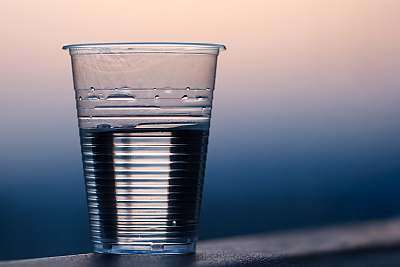# Cups to Ounces Calculator

Instructions: Use this calculator to convert cups to ounces, showing all the steps. Please type the number of cups you would like to convert in the form below:Type the number of cups:

## Using this Cups to Ounces Conversion Tool

Typically volume conversion is necessary when dealing with measurement coming from different countries.

In the tradition of the imperial units, measurements like cups, ounces and gallons are common, but in the metric tradition, measurements are centered around the liter.

So pretty much the same as we with lengths and heights, volumes require usual conversion. These conversion are quite simple in general, but it is not too easy to keep them all in the top of your head.## What is the Oz of 1 cup?

This is the first question that will allow to derive a general conversion rule. We know that 1 cup corresponds to 8 ounces, which is the starting point of the conversion process.

## How do you convert cups to ounces

All conversions of units are usually quite simple, the thing is that it may be hard to remember in the top of your head. One more effective way is to use a direct proportion to start the conversion.

## What are the steps for the conversion of cups into ounces

1. First, we observe that 1 cup is the same as 8 fluid ounces. This is your starting point
2. Now, given C cups. You want to know many fluid ounces O are equivalent to those C cups
3. The corresponding direct proportion that you need to use is given by $$\displaystyle \frac{1}{8} = \frac{C}{O}$$
4. Solving the proportion shown above for O, we get the following conversion formula from cups to ounces: $$O = 8 C$$

Observe that here cups refer to standard U.S. cups.

## Why would you want to convert cups to ounces

Many reasons, but perhaps you simply don't know what 2 cups is the equivalent in ounces. The problem with the traditional imperial system is that there are many volume units, and it is hard to know all of them.

For the metric system there is one basic unit, the liter, which is clear and well known, and the rest of units are derived from the liter, so it is easy to get idea of their magnitude.### Examples: Converting cups to fluid oz

Convert 1/4 cups to ounces

Solution: The problem is simple: we need to identify the passed number of cups and use the conversion formula to get the corresponding number of ounces. In this case we are given C = 1/4 cups. The formula for converting these cups into oz is

$O = 8 C$

Therefore, what is needed now is to plug the value of C = 1/4 in the conversion equation, so we get

$O = 8 C = 8 \times 1/4 = 2 \text{ oz}$

### Examples: Another cup to ounces conversion

What is 3/4 cup to oz

Solution: We have to apply the same procedure as what we applied above. We need to first identify the volume that needs to be converted. In this case we need to convert $$C = 0.75$$ cups into oz. Using the conversion formula leads to:

$O = 8 C = 8*3/4 = 6 \text{ oz}$

## Other volume conversions

So you get the point, right? If you want 4 cups to oz, you do O = 8*4 = 32 oz. Simple.

It is quite common to need to compute from cups to ounces, but is also common to convert from ounces to cups. The rationale is the same, you would solve for C instead in the proportionality equation.

Now, you may need even more help when dealing with two different system, as you may be interested in converting from ounces to liters, and vice versa, from liters to ounces, to make a bridge between the imperial and metric systems.

Other commonly used conversions deal with cups to quarts as well as quarts to gallons. You will find lots of tools that will give you volume conversions, but our calculator will show you all the steps of the process, you can help provide more clarity of the issue.